（给前端大全加星标，提升前端技能）

https://juejin.im/post/5cf61ed3e51d4555fd20a2f3

### 1. 熟悉的  `JSON.stringify()`

• 将 JSON object 存储到 localStorage 中；
• POST 请求中的 JSON body；
• 处理响应体中的 JSON 形式的数据；
• 甚至某些条件下，我们还会用它来实现一个简单的深拷贝；
• ……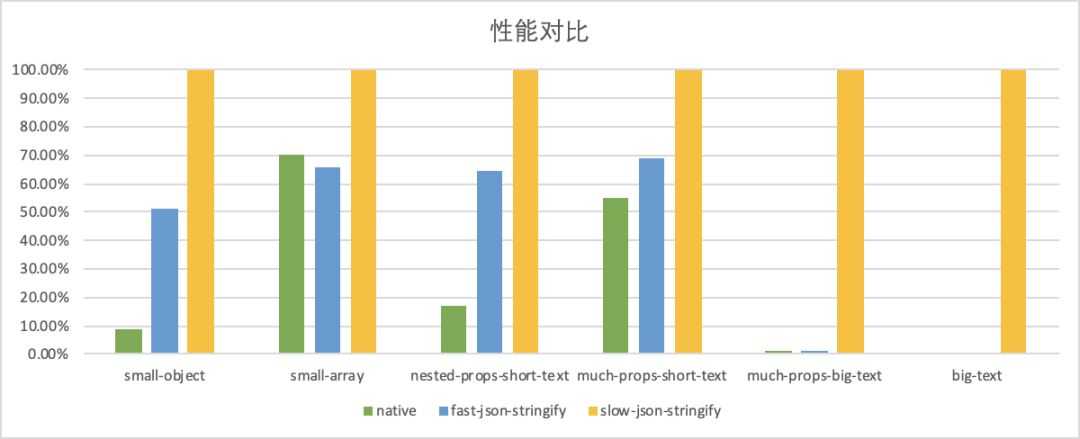### 2. 比  `stringify`  更快的  `stringify`

``````const obj = {

name: 'alienzhou',

status: 6,

working: true

};
``````

``````JSON.stringify(obj);

// {"name":"alienzhou","status":6,"working":true}
``````

• 键名不变
• 键值的类型一定

``````function myStringify(o) {

return (

'{"name":"'

+ o.name

+ '","status":'

+ o.status

+ ',"isWorking":'

+ o.working

+ '}'

);

}
``````

``````myStringify({

name: 'alienzhou',

status: 6,

working: true

});

// {"name":"alienzhou","status":6,"isWorking":true}

myStringify({

name: 'mengshou',

status: 3,

working: false

});

// {"name":"mengshou","status":3,"isWorking":false}
``````

• 需要先确定对象的结构信息；
• 根据其结构信息，为该种结构的对象创建“定制化”的  `stringify` 方法，其内部实际是通过字符串拼接生成结果的；
• 最后，使用该“定制化”的方法来 stringify 对象即可。

``````import faster from 'some_library_faster_stringify';

// 1. 通过相应规则，定义你的对象结构

const theObjectScheme = {

// ……

};

// 2. 根据结构，得到一个定制化的方法

const stringify = faster(theObjectScheme);

// 3. 调用方法，快速 stringify

const target = {

// ……

};

stringify(target);
``````

### 3. 如何生成“定制化”的方法

#### 3.1. fast-json-stringify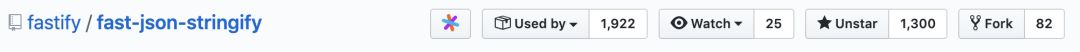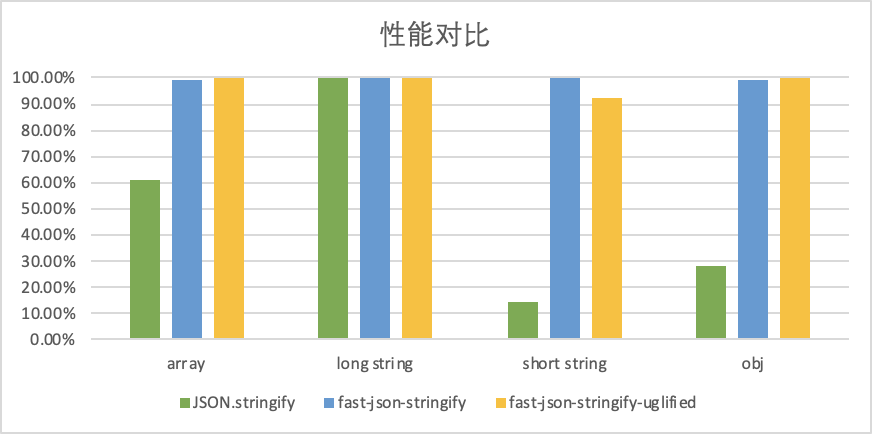###### 3.1.1. scheme 的定义方式

fast-json-stringify 使用了 JSON Schema Validation 来定义（JSON）对象的数据格式。其 scheme 定义的结构本身也是 JSON 格式的，例如对象

``````{

name: 'alienzhou',

status: 6,

working: true

}
``````

``````{

title: 'Example Schema',

type: 'object',

properties: {

name: {

type: 'string'

},

status: {

type: 'integer'

},

working: {

type: 'boolean'

}

}

}
``````

###### 3.1.2. stringify 方法的生成

fast-json-stringify 会根据刚才定义的 scheme，拼接生成出实际的函数代码字符串，然后使用 Function 构造函数在运行时动态生成对应的 stringify 函数。

``````var code = `

'use strict'

`

code += `

\${\$asString.toString()}

\${\$asStringNullable.toString()}

\${\$asStringSmall.toString()}

\${\$asNumber.toString()}

\${\$asNumberNullable.toString()}

\${\$asIntegerNullable.toString()}

\${\$asNull.toString()}

\${\$asBoolean.toString()}

\${\$asBooleanNullable.toString()}

`
``````

``````var accessor = key.indexOf('[') === 0 ? sanitizeKey(key) : `['\${sanitizeKey(key)}']`

switch (type) {

case 'null':

code += `

json += \$asNull()

`

break

case 'string':

code += nullable ? `json += obj\${accessor} === null ? null : \$asString(obj\${accessor})` : `json += \$asString(obj\${accessor})`

break

case 'integer':

code += nullable ? `json += obj\${accessor} === null ? null : \$asInteger(obj\${accessor})` : `json += \$asInteger(obj\${accessor})`

break

……
``````

``````"json += \$asString(obj['name'])"
``````

``````function \$asString(str) {

// ……

}

function \$asStringNullable(str) {

// ……

}

function \$asStringSmall(str) {

// ……

}

function \$asNumber(i) {

// ……

}

function \$asNumberNullable(i) {

// ……

}

/* 以上是一系列通用的键值转换方法 */

/* \$main 就是 stringify 的主体函数 */

function \$main(input) {

var obj = typeof input.toJSON === 'function'

? input.toJSON()

: input

var json = '{'

if (obj['name'] !== undefined) {

json += ','

}

json += '"name":'

json += \$asString(obj['name'])

}

// …… 其他属性(status、working)的拼接

json += '}'

return json

}

return \$main
``````

``````// dependencies 主要用于处理包含 anyOf 与 if 语法的情况

dependenciesName.push(code)

return (Function.apply(null, dependenciesName).apply(null, dependencies))
``````

#### 3.2. slow-json-stringify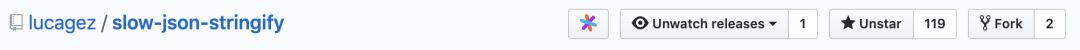slow-json-stringify 虽然名字叫 “slow”，但其实是一个 “fast” 的 stringify 库（命名很调皮）。

The slowest stringifier in the known universe. Just kidding, it’s the fastest (: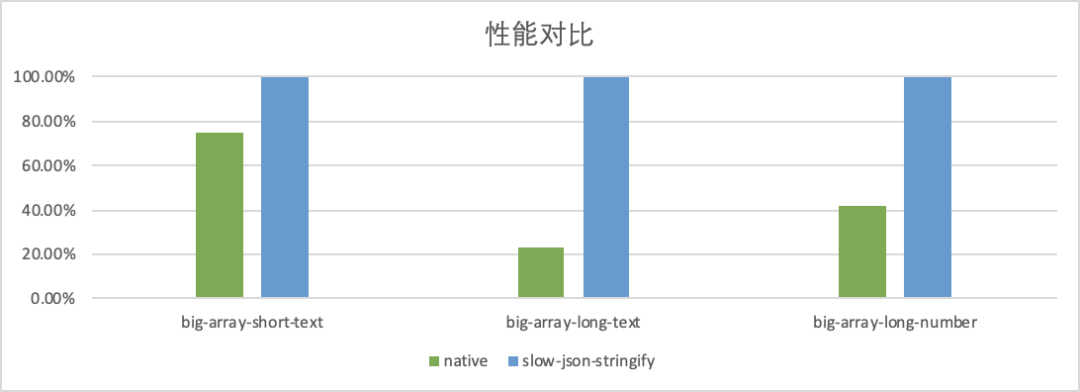###### 3.2.1. scheme 的定义方式

slow-json-stringify 的 scheme 定义更自然与简单，主要就是将键值替换为类型描述。还是上面这个对象的例子，scheme 会变为

``````{

name: 'string',

status: 'number',

working: 'boolean'

}
``````

###### 3.2.2. stringify 方法的生成

``````// scheme

{

name: 'string',

status: 'number',

working: 'boolean'

}

// 目标对象

{

name: 'alienzhou',

status: 6,

working: true

}
``````

scheme 和原对象的结构是不是很像？

``````let map = {};

const str = JSON.stringify(schema, (prop, value) => {

const isArray = Array.isArray(value);

if (typeof value !== 'object' || isArray) {

if (isArray) {

const current = value;

arrais.set(prop, current);

}

_validator(value);

map[prop] = _deepPath(schema, prop);

props += `"\${prop}"|`;

}

return value;

});
``````

``````const queue = [];

const chunks = str

.replace(regex, (type) => {

switch (type) {

case '"string"':

case '"undefined"':

return '"__par__"';

case '"number"':

case '"boolean"':

case '["array-simple"]':

case '[null]':

return '__par__';

default:

const prop = type.match(/(?<=").+?(?=")/);

queue.push(prop);

return type;

}

})

.split('__par__');
``````

``````// chunks

[

'{"name":"',

'","status":"',

'","working":"',

'"}'

]

// props

[

'name',

'status',

'working'

]
``````

### 4. 总结

• 开发者定义 Object 的 JSON scheme；
• stringify 库根据 scheme 生成对应的模版方法，模版方法里会对属性与值进行字符串拼接（显然，属性访问与字符串拼接的效率要高多了）；
• 最后开发者调用返回的方法来 stringify Object 即可。

### Tips

• 所有的 benchmark 只能作为一个参考，具体是否有性能提升、提升多少还是建议你在实际的业务中测试；
• fast-json-stringify 中使用到了 Function 构造函数，因此建议不要将用户输入直接用作 scheme，以防一些安全问题